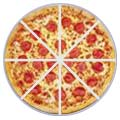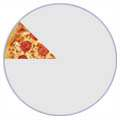# Multiplying Mixed Fractions

("Mixed Fractions" are also called "Mixed Numbers")

To multiply Mixed Fractions:

## Example: What is 138 × 3 ?

Think of Pizzas.138 is 1 pizza and 3 eighths of another pizza.

First, convert the mixed fraction (138) to an improper fraction (118):Cut the whole pizza into eighths and how many eighths do you have in total? 1 lot of 8, plus the 3 eighths = 8+3 = 11 eighths.

Now multiply that by 3:138 × 3 = 118 × 31 = 338 You have 33 eighths.

And, lastly, convert to a mixed fraction (only because the original fraction was in that form):33 eighths is 4 whole pizzas (4×8=32) and 1 eighth left over.

And this is what it looks like in one line:

138 × 3 = 118 × 31 = 338 = 418

## Another Example: What is 112 × 215 ?

Do the steps from above:

1. convert to Improper Fractions
2. Multiply the Fractions
3. convert the result back to Mixed Fractions### Step, by step it is:

Convert Mixed to Improper Fractions:

112 = 22+12 = 32

215 = 105+15 = 115

Multiply the fractions (multiply the top numbers, multiply bottom numbers):

32 × 115 = 3 × 112 × 5 = 3310

Convert to a mixed number

3310 = 3310

If you are clever you can do it all in one line like this:

112 × 215 = 32 × 115 = 3310 = 3310

## One More Example: What is 314 × 313 ?

Convert Mixed to Improper Fractions:

314 = 134

313 = 103

Multiply

134 × 103 = 13012

Convert to a mixed number:

13012 = 101012

And simplify:

101012 = 1056

Here it is in one line:

314 × 313 = 134 × 103 = 13012 = 101012 = 1056

## This One Has Negatives: What is −159 × −217 ?

Convert Mixed to Improper Fractions:

159 = 99 + 59 = 149
217 = 147 + 17 = 157

Then multiply the Improper Fractions (Note: negative times negative gives positive) :

−149 × −157 = −14 × −159 × 7 = 21063

We can simplify now. Here we use two steps, first by 7 (21 and 63 are both multiples of 7), then again by 3. But it could be done in one step by dividing by 21:

21063 = 309 = 103

Finally convert to a Mixed Fraction (because that was the style of the question):

103 = (9+1)3 = 93 + 13 = 313